## Cipher## Feb 24, 2017

### Supernova 1987A, Saturn/Pi coding of Supernova bullshit (do they really exist in reality?)

Here is the link to NASA's article that came out on 2/24/2017 - The Dawn of a New Era for Supernova 1987a
This story is a prime example of propaganda by the numbers, and these people actually call themselves scientistsFirst I should point out that they are bringing this up after 30th anniversary of discovery of this "Supernova" (in astrology Saturn's return takes 30 years (360 months) a lot like full circle (360°), it seems like these people are doing everything by the numbers of Saturn) - 30 years is 10,957 days (1331st prime number is 10,957 (11x11x11 = 1331) - 13 and 31 are also numbers associated with Saturn)
Three decades = 7+19+9+22+22 + 23+22+24+26+23+22+8 = 227 (Reverse Ordinal)
Note how their article starts with "Three decades" which has gematria connecting to circle (22/7 = 3.14), the coding of Pi and "inverse Pi" is very frequent with NASA and all other parts of mainstream
The supernova is called "1987a" which also connects to the circle which is the reason it's being reported by these numbers
Nineteen eighty seven A = 13+18+13+22+7+22+22+13 + 22+18+20+19+7+2 + 8+22+5+22+13 + 26 = 314 (Reverse Ordinal)
Twenty two divided by seven = 20+23+5+14+20+25 + 20+23+15 + 4+9+22+9+4+5+4 + 2+25 + 19+5+22+5+14 = 314 (Ordinal)This is a picture from the video in this article (Video link) , notice the 8 behind the circle of "Supernova". 8 is the symbol for infinity and also in astrology Saturn is the ruler of number 8 (note that 3.14 reduces to 8 as well)So this supernova was supposedly found on 2/23/1987 in the time of Pisces = 44 (S exception), Space = 44.... 2/23 stands for 223, a number coded over and over in mainstream media (Masonic = 223 Jewish, The Synagogue of Satan = 223, also 223 shows up in Revelation 13:18 where the number of man/beast (666) comes up)

Another reason for tor this Supernova to have Pi coding is the date numerology of it's "discovery"
2/23/1987 - 2+2+3 + 1+9+8+7 = 32Circle = 3+9+9+3+3+5 = 32 (Reduced)
2/23/1987 - (2) + (23) + 1+9+8+7 = 50Circle = 3+9+18+3+12+5 = 50 (Ordinal), Thirty two = 2+8+9+9+2+7 + 2+5+6 = 50 (Reduced)
2/23/1987 - (2) + (23) + (19) + (87) = 131 Supernova = 19+21+16+5+18+14+15+22+1 = 131 (Ordinal) - 32nd prime number is 131
2/23/87 - (2) + (23) + (87) = 112 - Supernova = 8+6+11+22+9+13+12+5+26 = 112 (Reverse Ordinal)
Circle = 24+18+9+24+15+22 = 112 (Reverse Ordinal)
Twenty third of February = 2+5+5+5+2+7 + 2+8+9+9+4 + 6+6 + 6+5+2+9+3+1+9+7 = 112 (Reduced)
Could there be a better date for the "discovery of Supernova"??
This coding is absolutely ridiculous... This is what science has become - pure propaganda by the numbers... Every part of society has been infiltrated by these spineless mongoloids and they make sure to put their fingerprint on everything you can find.I can't find anything for Oscar Duhalde
Shelton = 8+1+4+6+7+3+4 = 33 (Reverse Reduced)
Shelton = 19+8+5+12+20+15+14 = 93 (Ordinal)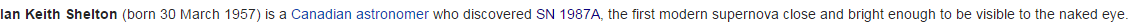Notice that he's born on 3/30 and he discovered this "Supernova" 330 days after his birthday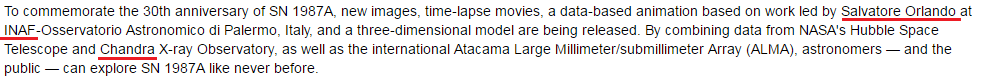Salvatore Orlando = 1+1+3+4+1+2+6+9+5 + 6+9+3+1+5+4+6 = 66 (Reduced)
INAF = 9+50+1+6 = 66 (English)
Thirty three = 2+8+9+9+2+7 + 2+8+9+5+5 = 66 (Reduced)

Chandra = 3+8+1+40+4+80+1 = 137 (Jewish) - 33rd prime number is 137
The significance of 137 probably comes from Golden Angle which can be seen in the way plants grow their leavesHere's a link that goes into all of that good stuff - Golden proportion in nature

There's some more stuff to this bullshit story but there's no reason to decode it any further since I already covered the key points.

### Movie Decode - The Circle - 227/47/33/156 - Release date - 4/28/2017

So I googled "Movies in 2017" to see what kind of propaganda they are working on for this year, and the one that immediately stood out was a movie called The Circle, when you see a name like this you can expect pretty beautiful coding

This movie is based on a book that was released in October 2013, the plot is basically foreshadowing the future of our world.Simply put, corporation called The Circle is trying to take absolute control of the world. They forcing people to use their full real name on all of their accounts and transactions and everything else. The whole world is heavily monitored by cameras and drones and all of the things they do online or anywhere are being recorded, analyzed and stored....so this book is really showing where they are taking us in the near future.

The trailer also does a pretty good job of showing how privacy isn't even a concept anymore and in the last part of the trailer you can hear the repeating voice saying: "They are watching you"

Dave Eggers = 23+26+5+22 + 22+20+20+22+9+8 = 177 (Reverse Ordinal)
In numerology you can drop the zero so 1051 becomes 151 connecting to circle through octal system
"Twenty two divided by seven" = 20+23+5+14+20+25 + 20+23+15 + 4+9+22+9+4+5+4 + 2+25 + 19+5+22+5+14 = 314 (Ordinal)
Dave Eggers is born on 3/12/1970 - 3+12+ 1+9+7+0 = 32 - "Circle" = 3+9+9+3+3+5 = 32 (Reduced)
Note how length of the trailer is 2:27
22/7 = 3.14 ... 7/22 = 0.318 ("inverse Pi") and this movie is coming out 318 days before Dave's birthday in 2018

The book was released on a date numerology corresponding to the writer and the title of the book
10/8/2013 - 10+8+20+13 = 51
Dave Eggers = 5+8+5+4 + 4+2+2+4+9+8 = 51 (Reverse Reduced)
The Circle = 100+8+5 + 3+9+80+3+20+5 = 233 (Jewish)

The Circle = 2+8+5 + 3+9+9+3+3+5 = 47 (Reduced) - 15th prime number
Released on a date with 15 numerology - 10/8/2013 = (1+0 +8+ 2+0+1+3 = 15
The movie is being released on April 28th 2017, 47 days after Dave's birthday - also 4/28 is 118th (119th) day in the year
Dave Eggers released the book 211 days after his birthday (47th prime number is 211)
33rd prime number is 137
The Circle was released in "October" = 6+3+2+6+2+5+9 = 33 (Reduced)
Cover of the book is in "Orange" = 6+9+1+5+7+5 = 33 (Reduced)
Dave Eggers was born in "Massachusetts" = 4+1+1+1+1+3+8+3+1+5+2+2+1 = 33 (Reduced)
Also he's born on "March Twelfth " = 13+1+18+3+8 + 20+23+5+12+6+20+8 = 137 (Ordinal)

The book was released on 8th of October
"Eighth of October" = 5+9+7+8+20+8 + 15+6 + 15+3+20+15+2+5+18 = 156 (Ordinal)
"Thirty three" = 20+8+9+18+20+25 + 20+8+18+5+5 = 156 (Ordinal)
Also the book was released 156 days before Dave's birthday in 2014 (156th prime number is 911)
9/11?
Nine Eleven = 5+9+5+5 + 5+3+5+4+5+5 = 51 (Reduced)
Dave Eggers = 51
9/11/2015 = 9+11+20+15 = 55 - the book was released 5 months and 5 days before Dave's birthday, "Los Angeles" = 3+6+10 + 1+5+7+5+3+5+10 = 55 (S Exception)

So measuring from 9/11/2015 to the release of the movie is 595 days
five hundred ninety five = 6+9+4+5 + 8+3+5+4+9+5+4 + 5+9+5+5+2+7 + 6+9+4+5 = 119 (Reduced)
five hundred ninety five = 21+18+5+22 + 19+6+13+23+9+22+23 + 13+18+13+22+7+2 + 21+18+5+22 = 322 (Reverse Ordinal)

Principal photography began 704 days after the release of the book - 33, 47 and 74 are the numbers that are always appearing together.
"Emma Watson" = 5+4+4+1 + 5+1+2+10+6+5 = 43 (S Exception)
"Tom Hanks" = 7+3+5 + 1+8+4+7+8 = 43 (Reverse Reduced)

Emma is playing Mae? Ema... Mae...
"Emma" = 5+30+30+1 = 66 (Jewish)
"Holland" = 8+15+12+12+1+14+4 = 66 (Ordinal)
"Holland" = 1+3+6+6+8+4+5 = 33 (Reverse Reduced)

Thirty three = 2+8+9+9+2+7 + 2+8+9+5+5 = 66 (Reduced)
Thirty three = 20+8+9+18+20+25 + 20+8+18+5+5 = 156 (Ordinal)
Tom Hanks is playing "Eamon" = 5+1+40+60+50 = 156 (English)
It's interesting how Eamon Bailey's gematria corresponds a bit to Dave Eggers
"Eamon Bailey" = 5+1+40+60+50 + 2+1+9+30+5+700 = 903 (English)
"Eamon Bailey" = 5+1+4+6+5 + 2+1+9+3+5+7 = 48 (Reduced)
"Dave Eggers" = 4+1+4+5 + 5+7+7+5+9+1 = 48 (Reduced)
"Dave Eggers" = 4+1+22+5 + 5+7+7+5+18+19 = 93 (Ordinal)

The Circle really represents America, since the circle coding began with the foundation of USA

## Feb 21, 2017

### "McCain steps up Trump criticism" - Here is why this is coming today 221/33

John McCain appeared on CNN  to "criticize" Trump today, and it's very interesting how they planned this event (CNN's - McCain Steps up Trump Criticism)
Trump criticism = 7+9+6+14+11 + 24+9+18+7+18+24+18+8+14 = 187 (Reverse Ordinal)
Lately there was a lot of 187 coding coming up in the news in relation to Trump, 187 is a very relevant number and a homicide code apart from that, the numbers that appear in this story could be interpreted to be assassination codes, however I will ignore that narrative completely, in my previous post I talked about 187 connections and other things relating to thatIn this video he actually mentions how the New World Order needs media to stay "FREE" in order to maintain this incredible system of "democracy" they established. He says: "Fundamental part of the New World Order is free press". Afterwards he even emphasizes how media being "FREE" is extremely important to him... What a joke.. These spineless morons truly have no shame or consciousness of any kind8/29/1936 - 8+2+9 + 1+9+3+6 = 38 - McCain = 5+6+6+8+9+4 = 38 (Reverse Reduced)
He's supposedly born in "Coco Solo" = 6+3+6+3 + 8+3+6+3 = 38 (Reverse Reduced)
"Panama Canal Zone" = 16+1+14+1+13+1 + 3+1+14+1+12 + 26+15+14+5 = 137 (Ordinal) - 33rd prime"Donald" = 4+50+40+1+20+4 = 119 (Jewish)
"Mr Trump" = 13+18 + 20+18+21+13+16 = 119 (Ordinal)
There's more connections that can be made to Trump from his name though
"John Sidney McCain III" = 10+15+8+14 + 19+9+4+14+5+25 + 13+3+3+1+9+14 + 9+9+9 = 193 (Ordinal)
44th prime number is 193 - Donald Trump is the 44th person to be the president but he is 45th term president also connecting to "John McCain"
John McCain = 1+6+8+5 + 4+3+3+1+9+5 = 45 (Reduced)

The length of the video is 2:57 (177 seconds) - and of course this moron is showing up here 177 days after his birthdayHere is how they measured to see when they want to have him show up to "criticize" Trump, they measured from Inauguration day to his birthday in 2017, which is 221 days a lot like this date 2/21/2017Also you can see how this date is coming 31 weeks and 4 days before his birthday a lot like Pi - 3.14, which is very relevant to Trump and a lot of the coding from the last few months, Trump won the election vote on 314th day of the year (11/9) and now here we have McCain(119) criticizing Trump on a day that leaves 314 days in the leap years"Pi" = 16+9 = 25 (Ordinal) - Super Bowl 51 took place on 2/5 - 2 months and 27 days after Trump won the election voteBut 2017 isn't a leap year, and there is also a connection to 313 of course, if we measure from 11/9/2016 to 2/21/2017, we see 3 months and 13 days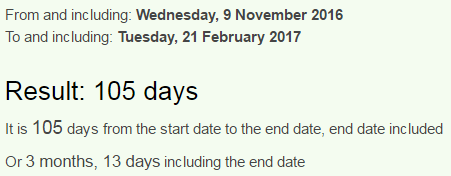Now we also see 105 days (Masonry = 105, Zionism = 105), which can be seen as 15 which connects to date numerology of 2/21/2017
2/21/2017 - 2+2+1+2+0+1+7 = 15 (47 is 15th prime number - President = 47) - "Trump" = 7+9+6+14+11 = 47 (Reverse Ordinal)

So they got 221 days by measuring from Inauguration to McCain's birthday and then they measured to 2/21 from Inauguration dayNote that this story came on a date numerology of 33 (2+21+2+0+1+7 = 33), 33 days after Inauguration day that had date numerology of 38 (1+20+17 = 38 - McCain = 38)
"John McCain" = 10+15+8+14 + 13+3+3+1+9+14 = 90 (Ordinal)
"Ninety" = 5+9+5+5+2+7 = 33 (Reduced)"Sen. John McCain" = 8+22+13 + 17+12+19+13 + 14+24+24+26+18+13 = 223 (Reverse Ordinal)
"The Arizona Republican" = 7+19+22 + 26+9+18+1+12+13+26 + 9+22+11+6+25+15+18+24+26+13 = 322 (Reverse Ordinal)
The Synagogue of Satan = 223, and 322 is the number of Skull & Bones...

## Feb 16, 2017

### 2/16 Time Cover 187 Trump assassination

Once again we see assassination codes for Trump... 10 days after he won the election I got a hint from a TV show about his assassination and it seems like a lot of the stuff is pointing to May Day in 2017... I will quickly explain how this connects to my prediction since I already covered a lot of the May 1st stuff in the last few monthsNothing to see here = 5+6+2+8+9+5+7 + 2+6 + 1+5+5 + 8+5+9+5 = 88 (Reduced)
Trump = 20+18+21+13+16 = 88 (Ordinal)
Nothing to see here = 4+3+7+1+9+4+2 + 7+3 + 8+4+4 + 1+4+9+4 = 74 (Reverse Reduced)
From 2/16 to May 1st is 74 days if we don't count the last day, but counting it gives us 2 months and 16 days...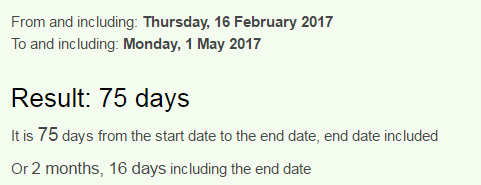Nothing to see here = 14+15+20+8+9+14+7 + 20+15 + 19+5+5 + 8+5+18+5 = 187 (Ordinal)
Nothing to see here except the big homicide code. Note the sum of divisors on 187... Homicide Code = 93 (Ordinal)216 (6x6x6)
May First = 78+6+150 + 36+54+108+114+120 = 666 (Sumerian)
May 1st also comes 135 days after Saturnalia in 2016, 93 in octal system is 135(+531) = 666
Saturnalia starts on "December Seventeenth" = 93 - S exception
May Day = 14+26+2 + 23+26+2 = 93 (Reverse Ordinal)Trump got 304 and Hillary got 227 electoral votes....... 304+227 = 531(+135) = 666
Super Bowl 51 came 2 months and 27 days after Trump won the election vote on 11/9/2016, and now from Super Bowl to May first there is 2 months and 27 days

This is a screenshot from Zach's post http://freetofindtruth.blogspot.rs/2017/02/47-74-88-97-187-1122-nothing-to-see.htmlThis year Saturnalia comes 187 days after Trump's birthday and it's interesting how Pope Francis is celebrating his birthday on Saturnalia, if Trump "dies" on 5/1 it will be 44 days before his 71st birthdayPope Francis = 60+50+60+5 + 6+80+1+40+3+9+90 = 404 (Jewish), he appeared on Super Bowl 51 on a date with 44 numerology
Saturnalia = 10+1+2+3+9+5+1+3+9+1 = 44 (S Exception)President = 47 - TIME = 20+9+13+5 = 47 (Ordinal), Trump = 7+9+6+14+11 = 47 (Reverse Ordinal), Trump = 100+80+200+30+60 = 470 (Jewish), Republican = 9+5+7+3+2+3+9+3+1+5 = 47 (Reduced)

2/16 leaves 318 days in the year which connects to Lucifer episode that came out on 11/14 (normally 318th day in the year), where Lucifer talks about Trump dying soon, note that the TV show went on a break until the first of May after releasing an episode named "A good day to die"Candy Morningstar = 6+8+4+5+2 + 5+3+9+4+9+4+2+8+7+8+9 = 93 (Reverse Reduced)
May Day = 14+26+2 + 23+26+2 = 93 (Reverse Ordinal)
The episode that came out on 11/14/2016 is called "Trip to stabby town" and Lucifer said Trump in the 44th second of the 38th minute (Kill = 44, Execution = 44, Death = 38, Killing = 38)Trump appears on TIME cover 3 months and 3 days after this episode came out, but there is interesting connection to his previous appearance on TIME which was 3 weeks and 3 days after this episodeThe last time Trump appeared on the cover of Time magazine was on Pearl Harbor day on 12/7/2016, and the date has absolutely matching date numerology with February 16th that comes 71/72 days later
12/7/2016 - 1+2+7 + 2+0+1+6 = 19    ---------  2/16/2017 - 2+1+6 + 2+0+1+7 = 19
12/7/2016 - (12) + (7) + 2+0+1+6 = 28  --------- 2/16/2017 - (2) + (16) + 2+0+1+7 = 28 (This is coming 28 days after Trump entered office)
12/7/2016 - (12) + (7) + (20) + (16) = 55  --------- 2/16/2017 - (2) + (16) + (20) + (17) = 55
12/7/16 -  (12) + (7) + (16) = 35  --------- 2/16/17 - (2) + (16) + (17) = 35Note that this is a span of 71 days and Trump could "die" 44 days before turning 71If we include the last day this Time cover is coming 72 days from Pearl Harbor day
Pearl Harbor day = 7+5+1+9+3 + 8+1+9+2+6+9 + 4+1+7 = 72 (Reduced)
Pearl Harbor day = 16+5+1+18+12 + 8+1+18+2+15+18 + 4+1+25 = 144 (Ordinal)
TIME = 100+9+30+5 = 144 (Jewish)
216 written out also connects to 144
Two one six = 20+23+15 + 15+14+5 + 19+9+24 = 144 (Ordinal)
Trump is also the 44th man to be the president

If he dies on May 1st it will also be 322 days from his last birthday (322 is the number of Skull and Bones and the reflection is "The Synagogue of Satan" = 223)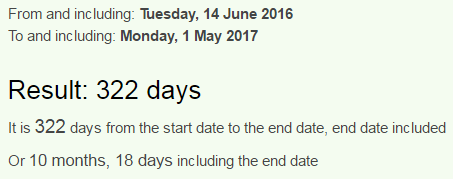May First = 5+8+2 + 3+9+9+8+7 = 51 (Reverse Reduced)
First of May = 6+9+9+1+2 + 6+6 + 4+1+7 = 51 (Reduced)
First of May = 21+18+9+8+7 + 12+21 + 14+26+2 = 138 (Reverse Ordinal)
Donald Trump = 4+15+14+1+12+4 + 20+18+21+13+16 = 138 (Ordinal)
We just saw Super Bowl 51... and I wouldn't be surprised for a second if they really have the date set for 5/1/2017.. There's so much crazy coding all around Trump.... I have at least 5 posts from the last 2 months covering a lot of Trump assassination stuff

### Daphnis Moon of Saturn, Classic case of Saturn worship

Realm of Daphnis - link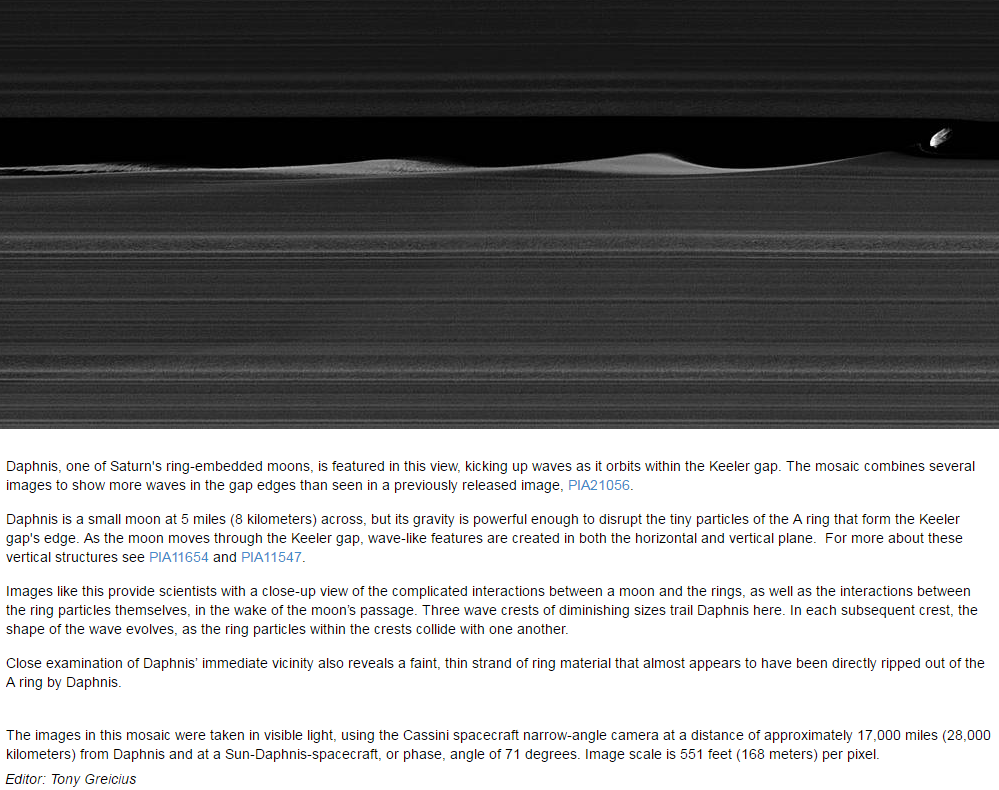Saturn = 19+1+20+21+18+14 = 93 (Ordinal)
When we look at Daphnis in Greek mythology we can see that it's associated with Pan (Pan is very much related to Saturn and it's easy to understand how they chose the name for this supposed moon of Saturn, note that another one of the 62 moons is named Pan) - In greek mythology both Pan and Daphnis are children of HermesBack to the NASA....The date of discovery has gematria matching up with Saturn
May Sixth = 13+1+25 + 19+9+24+20+8 = 119 (Ordinal)
(Saturn = 119 (F.B.), God Saturn = 119, Star of David = 119, All seeing eye = 119, Milky Way = 119)
Jet Propulsion Laboratory manages this mission for "NASA's Science Mission Directorate" - they manage the most of NASA "missions"
Jet Propulsion Laboratory = 1+5+2 7+9+6+7+3+3+10+9+6+5+3+1+2+6+9+1+2+6+9+7 = 119 (S Exception)
There's was another NASA article that I noted a few weeks back about Daphnis but I decided to wait and see if anything comes up, sure enough NASA never disappointsNASA released the "new photograph" a day later on 1/19  - Daphnis up close
The other reason 1/18 was chosen has to do with gematria of Daphnis
Daphnis = 23+26+11+19+13+18+8 = 118 (Reverse Ordinal)January Nineteenth = 8+8+4+6+8+9+2 + 4+9+4+4+7+4+4+4+7+1 = 93 (Reverse Reduced)Note how Daphnis is also known as Saturn XXXV (Saturn 35)
Daphnis = 4+1+7+8+5+9+1 = 35 (Reduced)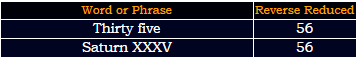Note how it was found on 5/6/2005
Daphnis is supposedly located in the Keeler Gap within the A Ring
Daphnis = 4+1+7+8+5+9+10 = 44 (S Exception)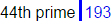Saturn's rings are named with letters from A-G
A Ring = 1 + 80+9+40+7 = 137 (Jewish)
This story comes on a date with 26 and 33 numerology (2+14+2+0+1+7 = 26, the story is released 26 days after "Daphnis Up close" article from 1/19)
2/14/17 = 2+14+17 = 33 (it was discovered on a date with sixteen numerology (5/6/05 = 16)The editor of this story is "Tony Greicius"Measuring from the date of discovery to the release of this story is 4302 days (432+234 = 666)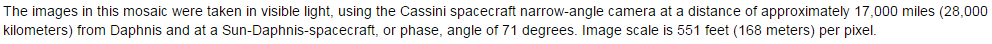They use 71 and 17 here, connecting to Daphnis ordinal sum and date numerology of 17 (2+1+4+2+0+1+7 = 17)
Daphnis = 4+1+16+8+14+9+19 = 71 (Ordinal)
"Image scale is 551 feet (168 meters) per pixel.

551+515+155 = 1221 (the same applies for 119, 227, 335 and 443)
168+186+618+681+816+861 = 3330

3330-1221 = 2109.
NASA is unbelievable... they truly make sure that everything is completely made up by the numbers... Talk about good science huh? This looks more like pure Saturn worship